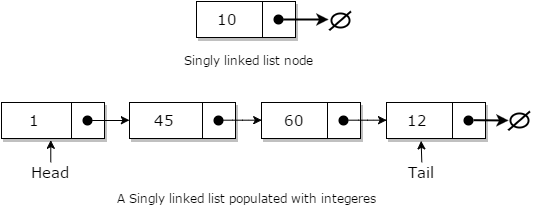The linked list or one way list is a linear set of data elements which is also termed as nodes. Here, the linear order is specified using pointers.

Each node is separated into two different parts:

• The first part holds the information of the element or node
• The second piece contains the address of the next node (link / next-pointer field) in this structure list.Linked lists can be measured as a form of high-level standpoint as being a series of nodes where each node has at least one single pointer to the next connected node, and in the case of the last node, a null pointer is used for representing that there will be no further nodes in the linked list. In the data structure, you will be implementing the linked lists which always maintain head and tail pointers for inserting values at either the head or tail of the list is a constant time operation. Randomly inserting of values is excluded using this concept and will follow a linear operation. As such, linked lists in data structure have some characteristics which are mentioned below:

• Insertion is O(1)
• Deletion is O(n)
• Searching is O(n)

Linked lists have a few key points that usually make them very efficient for implementing. These are:

• the list is dynamic and hence can be resized based on the requirement
• Secondly, the insertion is O(1).

Singly linked lists are one of the most primitive data structures you will learn in this tutorial. Here each node makes up a singly linked list and consists of a value and a reference to the next node (if any) in the list.## Insertion of Values in Linked List

In general, when people talk about insertion concerning linked lists of any form, they implicitly refer to the adding of a node to the tail of the list.

Adding a node to a singly linked list has only two cases:

• head = fi, in which case the node we are adding is now both the head and tail of the list; or
• we simply need to append our node onto the end of the list updating the tail reference appropriately

### Algorithm for inserting values to Linked List

• Pre: value is the value to be added to the list
• Post: value has been placed at the tail of the list
• n <- node(value)
• tail<- n
• else
• Next <- n
• tail <- n
• end if

Searching a linked list is straightforward: we simply traverse the list checking the value we are looking for with the value of each node in the linked list.

• value is the value to search for
• Post: the item is either in the linked list, true; otherwise false
• While n 6= fi and n.Value 6= value
• n <- n.Next
• end while
• if n = fi
• return false
• end if
• return true
• end Contains

Deleting a node from a linked list is straight-forward, but there are some cases that you need to account for:

• the list is empty; or
• the node to remove is the only node in the linked list; or
• we are removing the head node; or
• we are removing the tail node; or
• the node to remove is somewhere in between the head and tail; or
• the item to remove doesn't exist in the linked list

### Algorithm for Deletion

• value is the value to remove from the list
• Post: value is removed from the list, true; otherwise false
• return false
• end if
• If n.Value = value
• if the head = tail
• tail <- fi
• else
• end if
• return true
• end if
• while n.Next 6= fi and n.Next.Value 6= value
• n <- n.Next
• end while
• if n.Next 6= fi
• if n.Next = tail
• tail <- n
• end if
• // this is only case 5 if the conditional on line 25 was false
• Next <- n.Next.Next
• return true
• end if
• return false
• end Remove